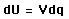# Charging a capacitor

## Homework Statement

A 3.00 MΩ resistor and a 1.00 μF capacitor are connected in series with an ideal battery of emf E = 4.00 V. At 1.00 sec after the connection is made, what is the rate at which

(a) the charge of the capacitor is increasing;
(b) energy is being stored in the capacitor;
(c) thermal energy is appearing in the resistor; and
(d) energy is being delivered by the battery?

## Homework Equations

VC=E(1-e-t/RC) ??

## The Attempt at a Solution

About the only thing I can work out is that the time constant (R*C=3 seconds).
I'm not sure how I work out rate of charge. but I can't see how any of the formulas I've looked at mention any of these things. Surely they wouldn't expect me to differentiate these equations myself (professor is always saying he wants us to do physics, not maths). What am I missing?

I assume for (a) I need to find out the instantaneous current? So I=E/R e-t/RC

4/3MΩ * e-1/3 = 0.955μA
Do I just express that in coulombs?

Is (b) just another way of asking (a) - or is this talking about something different?
(c) I've yet to google , and (d) I assume takes into account (b) and whatever is lost in (c).

For now, help with (a) would be nice.

Last edited by a moderator:

Just realised it only wants the rate, and not the rate of change of these things.
So (a) we are looking for current, so E/R e^(-t/RC)

= 4/3000000 * e^(-1/3) = 9.55uA ??

(b) energy I guess I use U = 1/2 CV^2 ?
(c) Relates to Power, so P=I^2*R
(d) can wait.

Is (b) just 1/2 QV, so 1/2 * 1/4 = 2 somethings ?

And (d) just QV, so 1x4 = 4.

NascentOxygen
Staff Emeritus
4/3MΩ * e-1/3 = 0.955μA
Do I just express that in coulombs?
current is coulombs/second, and coulombs/second are the units you'll be looking for to describe the rate that capacitor charge is changing

NascentOxygen
Staff Emeritus
Is (b) just 1/2 QV, so 1/2 * 1/4 = 2 somethings ?

And (d) just QV, so 1x4 = 4.
Energy stored on the capacitor is ½QV, but you are required to determine the rate-of-change of Energy

Nothing I can find. Can't even google myself a formula.

Closest guess would be -But I'm not even sure what dq are in this?

Last edited:
For (C) I'm assuming its P=I^2 R = 9.55x10^-6 * 3000000 = 2.74 x 10^-6 Watts ??

Last edited:
NascentOxygen
Staff Emeritus
For (C) I'm assuming its P=I^2 R = 9.55x10^-6 * 3000000 = 2.74 x 10^-6 Watts ??
I^2 R is resistor power, and power is Joules/second, so the formula is right. But is I = 9.55uA, or 0.955uA?

I^2 R is resistor power, and power is Joules/second, so the formula is right. But is I = 9.55uA, or 0.955uA?

Cheers, you're right, its 9.55 x 10^-7, so 0.955 uA.

Any hints for b and d ??

Is (b) requiring me to use ω CV Sin(ωt + pi/2) ???

Aside from that, I'm out of ideas.
Anyone ??

One last request for help. I have an hour and a half until I need to submit this.

NascentOxygen
Staff Emeritus
Is (b) just 1/2 QV, so 1/2 * 1/4 = 2 somethings ?
That's a good starting point, but remember you are looking for dU/dt.

There may be a shorter way than I'm thinking of, but if you start off with U(t) = ½Q(t).V(t) and differentiate you get (harking back to the derivative-of-a-product rule in Calculus 101)

dU/dt = ½Q.dV/dt + ½V.dQ/dt

Now, see whether you can change the dV/dt term on the RHS to a dQ/dt term, because if you can then simply substitute current for dQ/dt.

The equation Q = CV will be handy here. Indeed, it's a good equation to memorize, adding another arrow to your expanding physics quiver.That's a good starting point, but remember you are looking for dU/dt.

There may be a shorter way than I'm thinking of, but if you start off with U(t) = ½Q(t).V(t) and differentiate you get (harking back to the derivative-of-a-product rule in Calculus 101)

dU/dt = ½Q.dV/dt + ½V.dQ/dt

Now, see whether you can change the dV/dt term on the RHS to a dQ/dt term, because if you can then simply substitute current for dQ/dt.

The equation Q = CV will be handy here. Indeed, it's a good equation to memorize, adding it to your expanding physics quiver.Cheers, too late now, missed my deadline. I can't believe all I needed was the product rule (what I'm currently doing in maths .. oh man).
I'll play with this later in the week when I have a little free time.

Thanks for the help.